Scotty Hoag Programmer Portfolio   scotty.hoag@gmail.com  (209)-298-2622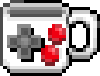# Lotka–Volterra / Predator-Prey Simulation

Anthro-196, Tutorial #3

The Theory

The Lotka-Volterra equation models populations of predator and prey species. We start out with a few initial assumptions:
1. The prey population finds ample food at all times.
2. The food supply of the predator population depends entirely on the prey populations.
3. The rate of change of population is proportional to its size.
4. During the process, the environment does not change in favour of one species and the genetic adaptation is sufficiently slow.
So, the prey have the potential to grow unbounded since they have infiinite food resources, while the predators can only grow while there is an ample supply of prey to eat. The number of births and deaths of either species increases as that species population increases. Lastly, we ignore effects of natural selection and evolution.

Let alpha be the birth rate of the prey species and beta be the death rate (Being eaten) of the prey. Let delta represent the birth rate of the predator species and gamma be the death rate of the predators. Then for prey species X and predator species Y, the relationship is defined as follows: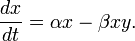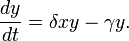So, the rate at which the prey species changes is equal to the number of births minus the number eaten. The rate at which the predator species is changing is the number of being born minus the number dying. Notice that beta does not necessarily equal delta, so the rate at which prey are born is not necessarily equal the number of prey being eaten. The effect produced by running a simulation with this behavior is that of two sine waves slightly out of phase with each other.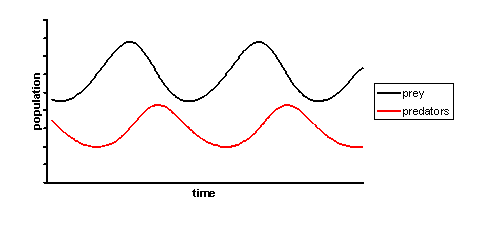As the predators increase in number, the prey decrease. As the prey population decreases, so does the population of predators that eat them. When the predator population dips low enough, the prey population begins to grow again. Thus, we have a cycle that continues unless the number of predators or prey reaches zero (extinction). If the prey go extinct, the predators will soon follow. If the predators go extinct, than the prey will grow in population without bound.

It is also possible that the two populations will reach an equilibrium point where the number of births and deaths cancel each other out for both species. By solving the Lotka-Volterra equations, we find that the equilibrium point occurs when the populations are as follows: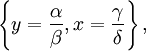That is, the two populations are in equilibrium when the number of predators is equal to the ratio of prey births to deaths and the number of prey is equal to the ratio of predator deaths to births.

Now that you know a little bit about the theory, let's look at the simulation code.

The Code

There are two Lotka-Volterra projects. If you examine them, they actually contain almost identical code, but running them produces very different results. Let's look at lotka_volterra_v02 first. This project runs a simulation based on the single-predator, single-prey dynamics mentioned above. There are several variables defined at the top of the file "lotka_volterra_v02.pde". The variable a represents alpha, b beta, d delta, and g gamma. Let's try setting them to the following values.

 ``float x_pop= 100.0; //population of preyfloat y_pop= 20.0; //population of predatorfloat z_pop= 0.0; //population of second predatorfloat a= 1.4;//growthrate of preyfloat b= 0.09;//deathrate of prey, being eaten and dyingfloat d= 0.001;//growthrate of predatorfloat g= 0.8;//deathrate of predatorfloat e= 0.0;//growthrate of second predatorfloat f= 0.0;//deathrate of second predatorfloat t = 0;//initial timefloat t_step = 0.01;//initial time stepfloat death_total = 0.0;//initial time stepfloat co2_total = 0.0;//all souls``

Run the simulation and see what you get. The green circles represent the prey species and the red circles represent predators. The brightness of the color is based on the population size, so larger populations produce brighter color intensity. You can see information about the exact population numbers printed in the window below the processing editor. The behaviour you should see is the oscillation effect predicted by Lotka and Volterra. The predator population will fluctuate between 3 and 40 members, while the prey population will fluctuate between 90 and 2800 members.

Now, try plugging in the values for the equilibrium equation mentioned above and set the variables x_pop and y_pop to your computed values. (You should get 800 for x_pop and 15.556 for y_pop) You should now observe that the predator and prey populations barely change at all. Now go back and try changing the initial population sizes and the birht and death rates. What kinds of behaviours do you observe? (Note that there are some safeguards in the code to prevent either species from going completely extinct.)

Now that you've seen the simulation running, let's take a look at the portion of the code that actually performs the Lotka-Volterra calculation. The code is in the lotka() function in the function.pde file. The important bits are in red.

 ``void lotka (){ float x_new = 0; float y_new = 0; x_new = (a*x_pop - b*x_pop*y_pop)*t_step+x_pop; y_new = (d*x_new*y_pop - g*y_pop)*t_step+y_pop; println("from Lotka"+x_new); //damper death_total = (x_new-x_pop)+(y_new-y_pop); if (x_new < 1.0){ x_pop= 1.0; } else { x_pop= x_new; } if (y_new < 1.0){ y_pop= 1.0; } else { y_pop= y_new; } co2_total = x_pop+y_pop; int prey = int(x_pop/10); int predator = int(y_pop); smooth(); for (int j = 0; j < prey; j++){ float dia = random(25); fill (0, j, 0); ellipse(random(x_screen), random(y_screen), dia, dia); } for (int i = 0; i < predator; i++){ float dia = random(25)+25; fill (i*12+128, 0, 0); ellipse(random(x_screen), random(y_screen), dia, dia); } }``

The first four red lines define a set of variables and set them to the new population size for x (Prey) and y (Predator) based on the Lotka-Volterra equation and the timestep value. Remember that the Lotka-Volterra equation produces a rate of change over time, so we have to multiply it by the timestep to get the actual change in population size. The two if statements after that just keep either species from going extinct by making sure that neither population gets too low.

Now let's take a look at lotka_volterra_v03_key. This project is very similar to lotka_volterra_v02, except that it includes a second predator that can eat both prey and the first predator. We now have three equations modeled thusly: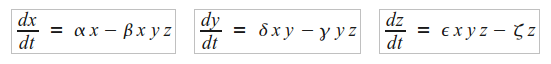So, the death rates of the prey and the first predator are now affected by this new super-predator species that can eat both of them. Likewise, the second predator's birth rate is dependant upon not only its own population, but upon the populations of the things that it can eat. Just as there were variables in lotka_volterra_v02 for the birth and death rates of our original prey and predator, there are variables in lotka_volterra_v03_key for our new species. The variable e represents epsilon (The new predator's birth rate) and f represents zeta (The new predator's death rate). Try the values below, then play around with the values and see what kinds of behaviours you observe.

 ``float x_pop= 100.0; //population of prey float y_pop= 20.0; //population of predatorfloat z_pop= 10.0; //population of second predatorfloat a= 1.4; //growthrate of prey float b= 0.09; //deathrate of prey, being eaten and dyingfloat d= 0.001; //growthrate of predatorfloat g= 0.8; //deathrate of predatorfloat e= 0.0001; //growthrate of second predatorfloat f= 0.9; //deathrate of second predatorfloat t = 0; //initial timefloat t_step = 0.01; //initial time stepfloat death_total = 0.0; //initial time stepfloat co2_total = 0.0; //all souls``

If you would like to add some flair to your predator-prey simulation, read on and I will show you how to replace the circles with images. We need to make a few changes. First, we need to find some images for our creatures and put them in the data folder of our project. Below are the images that I used.

 Prey Predator 1 Predator 2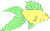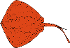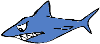Next, we need to load the images into our sketch. We do this by creating a PImage variable and then calling the loadImage function in the setup function. The lines I added are in violet. You will need to replace the filenames with whatever your images are named. Again, make sure your images are in the data folder!

 ``//These lines are outside of setup()``PImage prey1; PImage pred1;PImage pred2;void setup () { size(int(x_screen), int(y_screen)); `` //These lines are inside setup()`` prey1 = loadImage("prey1_small.png"); // <--- Replace filenames! pred1 = loadImage("pred1_small.png"); pred2 = loadImage("pred2_small.png");}``

Now in the functions.pde file, we need to locate the code that draws the creatures and change it from drawing circles to drawing images. I've outlined the prey's draw code in green, the first predator's draw code in red, and the second predator's draw code in blue.

 ``void triple_lotka (){ float x_new = 0; float y_new = 0; float z_new = 0; x_new = (a*x_pop - b*x_pop*y_pop*z_pop)*t_step+x_pop; y_new = (d*x_new*y_pop - g*y_pop*z_pop)*t_step+y_pop; z_new = (e*x_new*y_new*z_pop - f*z_pop)*t_step+z_pop; //println("from Triple Lotka: "+z_new); //damper death_total = (x_new-x_pop)+(y_new-y_pop); if (x_new < 1.0){ x_pop= 1.0; } else { x_pop= x_new; } if (y_new < 1.0){ y_pop= 1.0; } else { y_pop= y_new; } if (z_new < 1.0){ z_pop= 1.0; } else { z_pop= z_new; } co2_total = x_pop+y_pop; int prey = int(x_pop/10); int predator = int(y_pop); int predator2 = int(z_pop); smooth(); for (int j = 0; j < prey; j++){ float dia = random(25); fill (0, j, 0); ellipse(random(x_screen), random(y_screen), dia, dia); } for (int i = 0; i < predator; i++){ float dia = random(25)+25; fill (i*12+128, 0, 0); ellipse(random(x_screen), random(y_screen), dia, dia); } for (int k = 0; k < predator2; k++){ float dia = random(25)+25; fill (0, 0, (k*12)+128); ellipse(random(x_screen), random(y_screen), dia, dia); } }``

All that we have to do is comment out these lines and add in a few of our own.

 ``void triple_lotka (){ float x_new = 0; float y_new = 0; float z_new = 0; x_new = (a*x_pop - b*x_pop*y_pop*z_pop)*t_step+x_pop; y_new = (d*x_new*y_pop - g*y_pop*z_pop)*t_step+y_pop; z_new = (e*x_new*y_new*z_pop - f*z_pop)*t_step+z_pop; //println("from Triple Lotka: "+z_new); //damper death_total = (x_new-x_pop)+(y_new-y_pop); if (x_new < 1.0){ x_pop= 1.0; } else { x_pop= x_new; } if (y_new < 1.0){ y_pop= 1.0; } else { y_pop= y_new; } if (z_new < 1.0){ z_pop= 1.0; } else { z_pop= z_new; } co2_total = x_pop+y_pop; int prey = int(x_pop/10); int predator = int(y_pop); int predator2 = int(z_pop); smooth(); for (int j = 0; j < prey; j++){ //float dia = random(25); //fill (0, j, 0); //ellipse(random(x_screen), random(y_screen), dia, dia); image(prey1, random(x_screen), random(y_screen)); } for (int i = 0; i < predator; i++){ //float dia = random(25)+25; //fill (i*12+128, 0, 0); //ellipse(random(x_screen), random(y_screen), dia, dia); image(pred1, random(x_screen), random(y_screen)); } for (int k = 0; k < predator2; k++){ //float dia = random(25)+25; //fill (0, 0, (k*12)+128); //ellipse(random(x_screen), random(y_screen), dia, dia); image(pred2, random(x_screen), random(y_screen)); } }``

And that's it! You should now have pretty sharks and fish swimming around. Have fun with your simulation.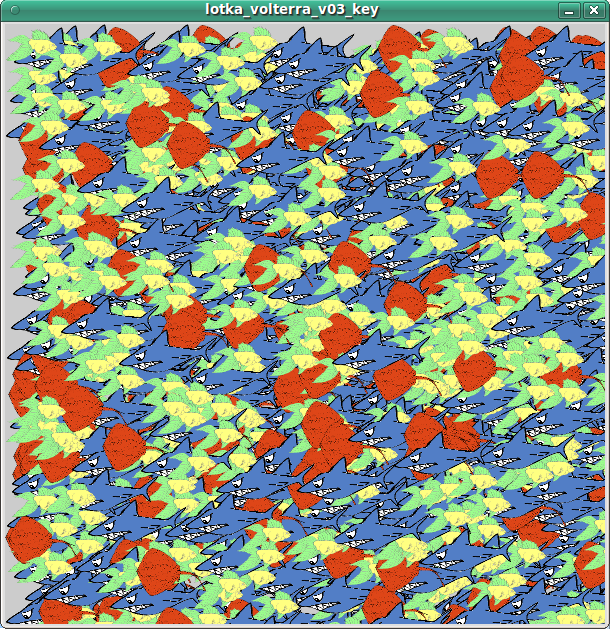All information comes from the Wikipedia article. Wile E. Coyote and the Road Runner are properties of Warner Bros. Entertainment. No animals were harmed in the creation of this tutorial.